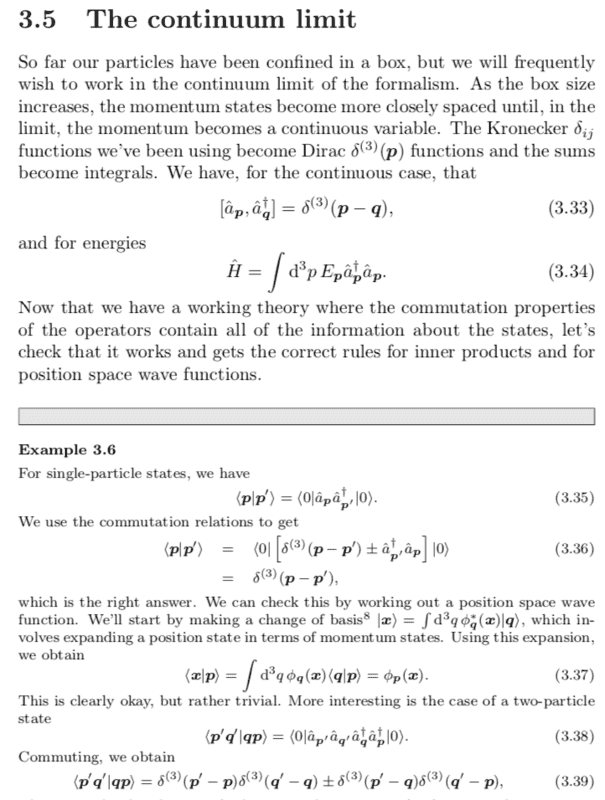# Operations regarding tensor-product states

• I
WWCY
TL;DR Summary
Confusion regarding the inner product of tensor-product states
I have a question on tensor-product states that I'd like to ask, thanks in advance!

1. The basis vector of a two-particle state can be written as ##|\mu_i \rangle |\nu_j \rangle## for orthonormal vectors ##|\mu_i \rangle, |\nu_j \rangle## spanning their single-particle Hilbert spaces. The inner product of basis vectors can be written as ##\langle \mu_{i'}|\langle \nu_{j'}|\mu_i \rangle |\nu_j \rangle = \delta_{i,i'} \delta_{j,j'}##

Does this hold for kets with continuous degrees of freedom? Eg momentum ##\langle p',q' |p,q \rangle = \delta(p-p') \delta(q-q')##. After reading Blundell's book on QFT however (screenshot provided below), I attempted to use creation and annihilation operators to obtain the above identity, but what I kept obtaining was ##\langle p',q' |p,q \rangle = \langle 0|\hat{a_{p'}}\hat{a_{q'}}\hat{a_{p}}^\dagger\hat{a_{q}}^\dagger|0\rangle = \delta(p-p') \delta(q-q') + \delta(q-p')\delta(p-q')##.•